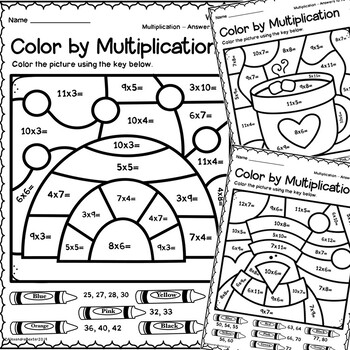# Winter Color by Multiplication Worksheets | Winter Fast Finishers3rd - 4th, Homeschool
Subjects
Standards
Resource Type
Formats Included
• PDF
Pages
16 (8 Worksheets & 8 Answer Keys)
##### Also included in
1. Check out these color by number worksheets for addition, subtraction, multiplication, and division. These no prep math facts activities are perfect for your winter math lessons and math centers. These color by code worksheets are also idea for morning work, homework, or early finishers! Students wil
Price \$8.50Original Price \$12.00Save \$3.50
2. These color by code / color by number activities are no prep! These color by multiplication worksheets are perfect for your math lessons and centers. The printables are Spring, Summer, Autumn Fall, and Winter themed and are ideal for morning work, homework, or early finishers!Students will enjoy mul
Price \$8.50Original Price \$12.00Save \$3.50
3. This mega bundle is a selection of color by code / color by number worksheets for addition, subtraction, multiplication, and division. These no prep activities are perfect for your Spring, Summer, Fall, or Winter math lessons and math centers. These activities are perfect for 3rd grade math fact pra
Price \$33.50Original Price \$48.00Save \$14.50

### Description

This resource is a selection of Color by Code / Color by Number: Multiplication worksheets. These no prep activities are great for Math lessons/centers during Winter. Students will enjoy multiplying using these engaging printables.

What is included?

8 different mystery pictures and matching answer keys. The worksheets increase in difficulty. For example, the first one has answers that go up to 12 with sums like 2x5 or 3x4. The last worksheet has answers that go up to 96 with sums like 10x9 or 8x8. This is great for multiple year levels and differentiation.

When/how to use this resource:

• Whole group lessons
• Small group work (math centers)
• Independent math fact practice
• Early finishers
• Morning work
• Homework
• Assessment

Reasons to buy this AMAZING product:

• It’s a time saver – simply print and go!
• It’s a bargain!
• It’s versatile – students in second to fifth grade could try this worksheet, depending on their capabilities
• It’s Common Core aligned
• It’s engaging – student friendly pictures, full of wonderful clip art (which kids will love coloring in)!

Enjoy this resource! Don't forget to follow my store for more awesome products and rate this product for your TPT credits :)

Let's Connect!

Pinterest

Instagram

Grab the bundles -

Subtraction Bundle

Multiplication Bundle

Division Bundle

Seasons -

Spring Bundle

Summer Bundle

Autumn Fall Bundle

Winter Bundle

Total Pages
16 (8 Worksheets & 8 Answer Keys)
Included
Teaching Duration
N/A
Report this resource to TpT
Reported resources will be reviewed by our team. Report this resource to let us know if this resource violates TpT’s content guidelines.

### Standards

to see state-specific standards (only available in the US).
Interpret products of whole numbers, e.g., interpret 5 × 7 as the total number of objects in 5 groups of 7 objects each. For example, describe a context in which a total number of objects can be expressed as 5 × 7.
Determine the unknown whole number in a multiplication or division equation relating three whole numbers. For example, determine the unknown number that makes the equation true in each of the equations 8 × ? = 48, 5 = __ ÷ 3, 6 × 6 = ?.
Apply properties of operations as strategies to multiply and divide. Examples: If 6 × 4 = 24 is known, then 4 × 6 = 24 is also known. (Commutative property of multiplication.) 3 × 5 × 2 can be found by 3 × 5 = 15, then 15 × 2 = 30, or by 5 × 2 = 10, then 3 × 10 = 30. (Associative property of multiplication.) Knowing that 8 × 5 = 40 and 8 × 2 = 16, one can find 8 × 7 as 8 × (5 + 2) = (8 × 5) + (8 × 2) = 40 + 16 = 56. (Distributive property.)
Fluently multiply and divide within 100, using strategies such as the relationship between multiplication and division (e.g., knowing that 8 × 5 = 40, one knows 40 ÷ 5 = 8) or properties of operations. By the end of Grade 3, know from memory all products of two one-digit numbers.
Interpret a multiplication equation as a comparison, e.g., interpret 35 = 5 × 7 as a statement that 35 is 5 times as many as 7 and 7 times as many as 5. Represent verbal statements of multiplicative comparisons as multiplication equations.

### Questions & Answers

Teachers Pay Teachers is an online marketplace where teachers buy and sell original educational materials.